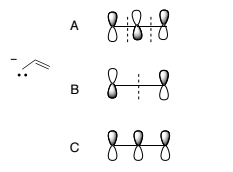# Problem: Consider the Molecular Orbitals (MO’s) of the allyl anion. Which are the HOMO and LUMO?  1) HOMO = B, LUMO = C 2) HOMO = B, LUMO = A 3) HOMO = C, LUMO = A 4) HOMO = A, LUMO = C 5) HOMO = C, LUMO = B

🤓 Based on our data, we think this question is relevant for Professor Ayres' class at UC.

###### Problem Details

Consider the Molecular Orbitals (MO’s) of the allyl anion. Which are the HOMO and LUMO?

1) HOMO = B, LUMO = C

2) HOMO = B, LUMO = A

3) HOMO = C, LUMO = A

4) HOMO = A, LUMO = C

5) HOMO = C, LUMO = B